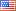# Rheological articles

## How to Measure Polymer`s Apparent Melt Density using a Melt Indexer

Method A/B is a test that can be performed in a melt flow rate tester in order to calculate the value of the polymer`s melt density at a specific temperature. In a method A/B test, both a “method A” test and “method B” test are performed on the same charge of material. The melt flow rate derived from method A, is equated to the method B flow equation and solved for apparent melt density. The value in doing this test is obtaining a valid apparent melt density which can later be used in a solo method B test (there is no manual cuts of extrudate or weighing in method B) to achieve results equivalent to method A (operator must make manual cuts and weigh sample in method A).

#### 07.09.2019

Method A/B is a test that can be performed in a melt flow rate tester in order to calculate the value of the polymer`s melt density at a specific temperature. In a method A/B test, both a “method A” test and “method B” test are performed on the same charge of material. The melt flow rate derived from method A, is equated to the method B flow equation and solved for apparent melt density. The value in doing this test is obtaining a valid apparent melt density which can later be used in a solo method B test (there is no manual cuts of extrudate or weighing in method B) to achieve results equivalent to method A (operator must make manual cuts and weigh sample in method A).

The mass melt flow rate from Method A is calculated based on the mass of the extrudate(s) as equation below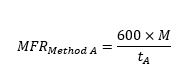where  (g) is the mass in grams of material collected over time  (sec). The value of “600” is taken into account in order to convent the unit of MFR to g/10 minMass measurement of MFR using a Dynisco LMI5500 series

The melt volume flow rate (MVR) from Method B is calculated as equation below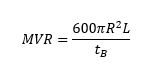where R is standard piston radios (R= 0.477 cm) , tB is the time of the piston travel for distance of . Based on ASTM D1238  can be set to be 0.635 +/- 0.025 cm or 2.540 +/- 0.025 cm measuring range depending on the expected MFR of the samples. The value of “600” is taken into account in order to convent the unit of MVR to cm3/10min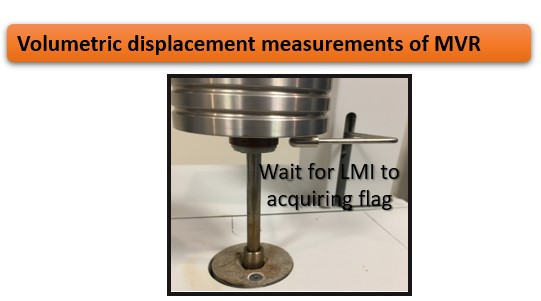Volumetric displacement measurement of MVR using a Dynisco LMI5500

The ratio of the two values (MFR from Method A and MVR from Method B) is a measure of the melt density of the polymer in g/cm3 as below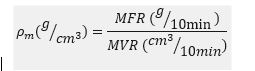Figure 1 shows the results for apparent melt density for two different EVA materials using a Dynisco LMI series melt indexer (test temperature: 190 C, weight: 2.16 kg). For this test 3 cutes were collected within 1 minute time interval for Method A part as well as acquiring 3 flags with flag length of 6.35 mm for Method B part.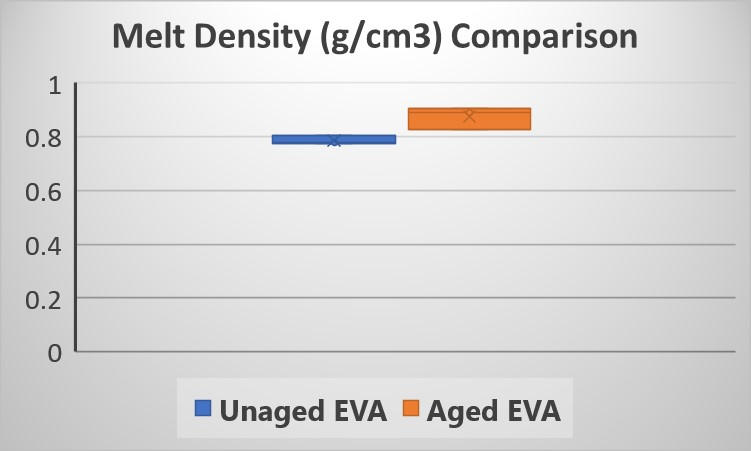Fig. 1. Apparent melt density results from Dynisco LMI series.

This apparent melt density definition forces the two test methods to agree. It is called an “apparent” melt density since it is actually a correlation coefficient which forces Method A and Method B to agree. If there were no leakage past the plunger tip and the extrudate were bubble free and few other minor factors were taken into consideration, then a true melt density could be assessed. This apparent melt density value can be used for Method B tests to obtain MFR values without manual cutting and weighing of the samples. Please note that the melt density of the polymer is a function of temperature. Also, fillers, reinforcing agent. etc. tend to change the melt density of the material